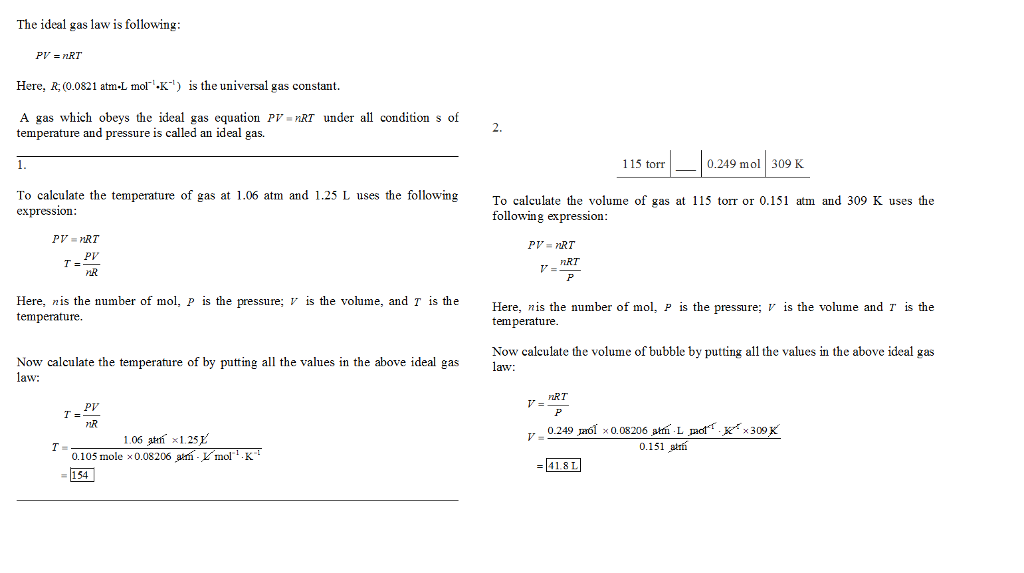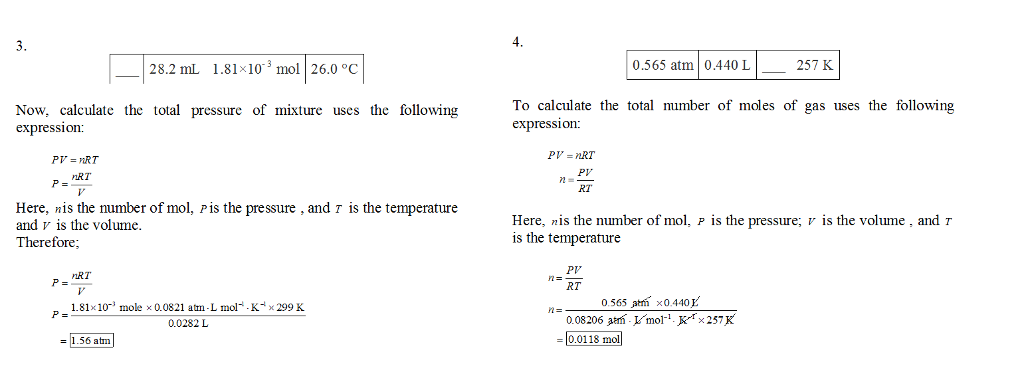In: Chemistry

# Use the ideal gas law to complete the table: P V n T 1.06 atm 1.25...

Use the ideal gas law to complete the table:

 P V n T 1.06 atm 1.25 L 0.105 mol ___ 115 torr ___ 0.249 mol 309 K ___ 28.2 mL 1.81×10−3 mol 26.0 ∘C 0.565 atm 0.440 L ___ 257 K

## Solutions

##### Expert Solution## Related Solutions

##### For an ideal gas, consider the molar volume Vm = (V/n) = Vm(T,P). In other words,...
For an ideal gas, consider the molar volume Vm = (V/n) = Vm(T,P). In other words, the molar volume is a function of temperature and pressure. a) Write the total differential dVm. b) Show that dVm is exact. c) Derive an expression for the differential work dw performed in a reversible process by expansion/compression of the gas. d) Show that dw is inexact. e) What is the thermodynamic significance of having an exact differential?
##### The ideal gas law can be stated as P V = nRT where P = pressure...
The ideal gas law can be stated as P V = nRT where P = pressure in Pascals (Pa) V = volume in cubic meters (m3 ) n = number of moles in moles (mol) R = gas constant = 8.3145m3 Pa / ( K · mol ) T = temperature in Kelvin (K). Using MATLAB or Octave, Write a script that prompts the user for the pressure, the volume, and the temperature; storing each in a variable. The script...
##### The ideal gas law PV=nRT relates pressure P, volume V, temperature T, and number of moles...
The ideal gas law PV=nRT relates pressure P, volume V, temperature T, and number of moles of a gas, n. The gas constant R equals 0.08206 L⋅atm/(K⋅mol) or 8.3145 J/(K⋅mol). The equation can be rearranged as follows to solve for n: n=PVRT This equation is useful when dealing with gaseous reactions because stoichiometric calculations involve mole ratios. Part A When heated, calcium carbonate decomposes to yield calcium oxide and carbon dioxide gas via the reaction CaCO3(s)→CaO(s)+CO2(g) What is the mass...
##### Suppose 1.0 mol of an ideal gas is initially at P=4.0 atm and T=400 K. It...
Suppose 1.0 mol of an ideal gas is initially at P=4.0 atm and T=400 K. It is expanded irreversibly and adiabatically against a constant pressure of 1.0 atm until the volume has doubled. (a) Calculate the final volume of the gas. (b) Calculate w, q, and energy change ΔU of this process, in joules. (c) Calculate the final temperature of the gas. (d) Calculate the entropy change ΔS of the ideal gas in the process. (e) What is the entropy...
##### For 1 mol of an ideal gas, Pexternal = P = 1 atm. The temperature is...
For 1 mol of an ideal gas, Pexternal = P = 1 atm. The temperature is changed from 125ºC to 25.0ºC, and CV,m = 3/2R. Calculate (all units are J) q= , w= , ∆U= , and ∆H= . Please enter your answers with 2 decimals in E notation, such as 2.33E4 (=23345). If the answer is negative, please do not forget the negative sign. If answer is zero, please just enter 0 without decimal.
##### Use Charles's law to complete the table. (Assume pressure and number of moles of gas to...
Use Charles's law to complete the table. (Assume pressure and number of moles of gas to be constant.) Part V1 T1 V2 T2 A 119 L 10.2 ∘C __ 106.4 ∘C B __ 135 K 173 L 314 K C 2.15 L 16.4 ∘C 2.23 L __ D 15.6 cm3 __ 18.2 cm3 10.1 ∘C A&B Express the volume in liters to three significant figures. C&D Express the temperature in degrees Celsius to three significant figures.
##### One mole of an ideal gas in an initial state P = 10atm, V = 5L,...
One mole of an ideal gas in an initial state P = 10atm, V = 5L, is taken reversibly in aclockwise direction around a circular path given by (V − 10)^2 + (P − 10)^2 = 25. Computethe amount of work done by the gas and the change in internal energy.
##### Two moles of an ideal gas originally at T = 400 K and V = 40...
Two moles of an ideal gas originally at T = 400 K and V = 40 L undergo a free expansion; ?2 = 10?1 . Find (a) the entropy change of the gas, and (b) the entropy change of the universe?
##### 1 Ideal Gas Law The ideal gas law is familiar to anyone who has taken a...
1 Ideal Gas Law The ideal gas law is familiar to anyone who has taken a college chemistry course: P V = νRT. This problem will show you why the ideal gas law has this form. We can arrive at this expression just by using classical mechanics! Consider a box of volume V containing N particles, each having mass m, that are moving horizontally with average speed v. The particles bounce back and forth between the end walls of the...
##### Calculus dictates that (∂U/∂V) T,Ni = T(∂S/∂V)T,Ni – p = T(∂p/∂T)V,Ni – p (a) Calculate (∂U/∂V)...
Calculus dictates that (∂U/∂V) T,Ni = T(∂S/∂V)T,Ni – p = T(∂p/∂T)V,Ni – p (a) Calculate (∂U/∂V) T,N for an ideal gas [ for which p = nRT/V ] (b) Calculate (∂U/∂V) T,N for a van der Waals gas [ for which p = nRT/(V–nb) – a (n/V)2 ] (c) Give a physical explanation for the difference between the two. (Note: Since the mole number n is just the particle number N divided by Avogadro’s number, holding one constant is equivalent...